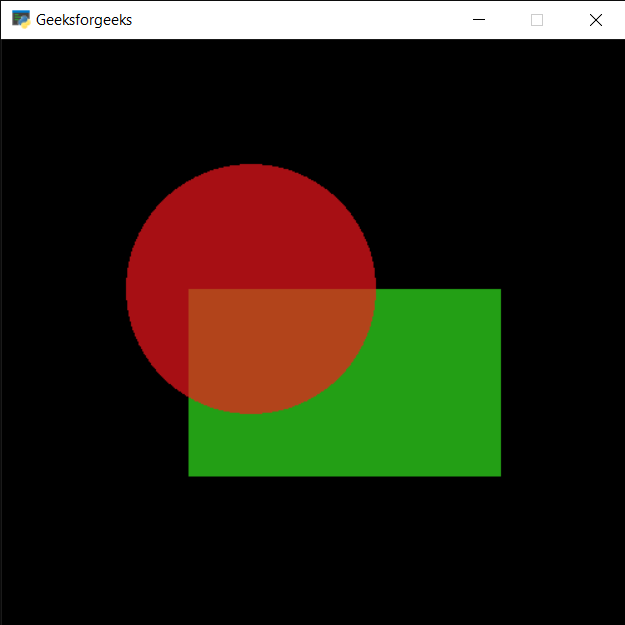# PYGLET – Shape X, Y Co-ordinates

• Last Updated : 09 Jul, 2021

In this article we will see how we can access the X & Y co-ordinates of the shape in PYGLET module in python. Pyglet is easy to use but powerful library for developing visually rich GUI applications like games, multimedia etc. A window is a “heavyweight” object occupying operating system resources. Windows may appear as floating regions or can be set to fill an entire screen (fullscreen). A shape is the form of an object or its external boundary, outline, or external surface, as opposed to other properties such as color, texture or material type. Many shapes are drawn with the help of shapes module of pyglet. X, Y co-ordinate specify the location of the shape in the window.
We can create a window with the help of command given below

```# creating a window
window = pyglet.window.Window(width, height, title)```

In order to create window we use x and y attribute with the shape
Syntax : shape.x and shape.y
Argument : It takes no argument
Return : It returns integer or float

Below is the implementation

## Python3

 `# importing pyglet module``import` `pyglet` `# importing shapes from the pyglet``from` `pyglet ``import` `shapes` `# width of window``width ``=` `500``  ` `# height of window``height ``=` `500``  ` `# caption i.e title of the window``title ``=` `"Geeksforgeeks"``  ` `# creating a window``window ``=` `pyglet.window.Window(width, height, title)` `# creating a batch object``batch ``=` `pyglet.graphics.Batch()`  `# properties of rectangle``# co-ordinates of rectangle``co_x ``=` `150``co_y ``=` `150` `# width of rectangle``width ``=` `250` `# height of rectangle``height ``=` `150` `# color = green``color ``=` `(``50``, ``225``, ``30``)` `# creating a rectangle``rec ``=` `shapes.Rectangle(co_x, co_y, width, height, color ``=` `color, batch ``=` `batch)` `# changing opacity of the rect1``# opacity is visibility (0 = invisible, 255 means visible)``rec.opacity ``=` `180`  `# creating another rectangle with properties``# x, y co ordinate : 50, 250``# width, height of rectangle : 300, 200``# color = red``color ``=` `(``255``, ``25``, ``25``)` `# properties of circle``# co-ordinates of circle``circle_x ``=` `200``circle_y ``=` `300` `# size of circle``# color = green``size_circle ``=` `100` `# creating a circle``circle ``=` `shapes.Circle(circle_x, circle_y, size_circle, color ``=``(``250``, ``22``, ``30``), batch ``=` `batch)` `# changing opacity of the circle1``# opacity is visibility (0 = invisible, 255 means visible)``circle.opacity ``=` `170`  `# window draw event``@window``.event``def` `on_draw():``    ` `    ``# clear the window``    ``window.clear()``    ` `    ``# draw the batch``    ``batch.draw()``    ` `    `  `# accessing x, y co-ordinate of rectangle``value_rec_x ``=` `rec.x``value_rec_y ``=` `rec.y` `# printing value``print``(``"Rectangle : "``, end ``=` `"")``print``(value_rec_x, value_rec_y)` `# accessing x, y co-ordinate of circle``value_cir_x ``=` `circle.x``value_cir_y ``=` `circle.y` `# printing value``print``(``"Circle : "``, end ``=` `"")``print``(value_cir_x, value_cir_y)`   `# run the pyglet application``pyglet.app.run()`

Output :```Rectangle : 150 150
Circle : 200 300```

My Personal Notes arrow_drop_up